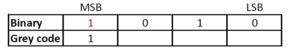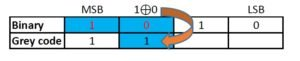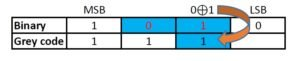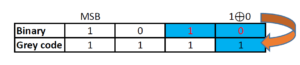Use the below online conversion tool to convert any binary to gray code:

 Binary Number Gray Code

Also Try:

## Binary number

Binary numbering system uses only two symbols 0 and 1. Each digits of a binary number is referred to as bits. Binary system is also known as base -2 system. Each digit is represented by the increasing power of 2 from the LSB (Least Significant Bit). Binary system is the heart of digital electronics and is used for information flow. In digital electronics, 0 and 1 are used to denote logic states, high and low. Arithmetic operations are also possible in binary system.

## Gray code

Gray code, also known as reflected binary code, is a code having digits 0 and 1. Gray code do not have place value for its digits. Any successive codes in Gray code system have only one bit changes.

## Binary to gray code conversion can be made easy with an example:

### Let us convert a binary value of 1010 to gray code:

Step 1: The MSB (Most Significant Bit) of a gray code and binary code will be the same.Step 2: The next digit of gray code will be the EXOR of the MSB and the digit right to the MSB of the binary code.Step 3: Similarly EXOR the digit in place and the previous digit of binary code to obtain the next digit of gray code.Step 4: Repeat the previous step till the LSB of gray code is found.## Decimal to binary to gray conversion table

DecimalBinaryGray
000000000
100010001
200100011
300110010
401000110
501010111
601100101
701110100
810001100
910011101
1010101111
1110111110
1211001010
1311011011
1411101001
1511111000

Also Try: